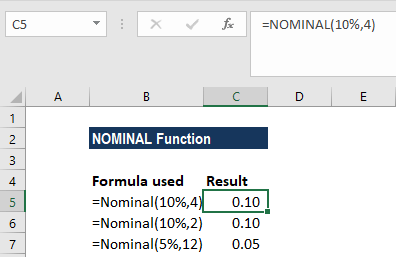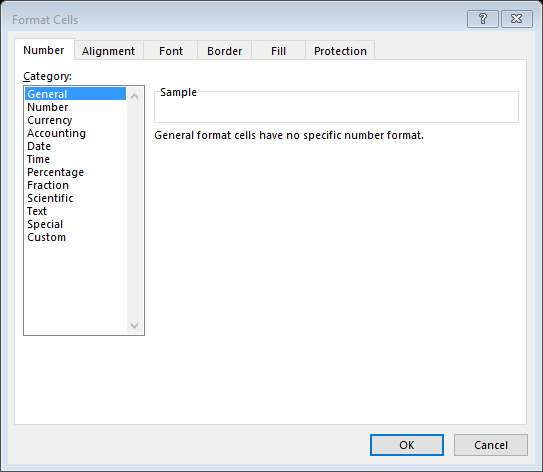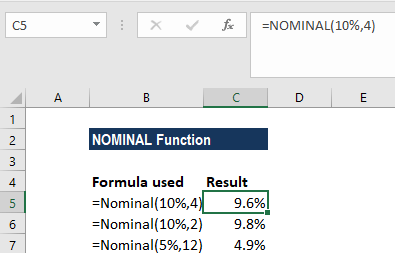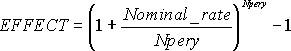# NOMINAL Function

Returns the nominal annual interest rate

## What is the NOMINAL Function?

The NOMINAL function is categorized under Excel Financial functions. The function will return the nominal annual interest rate when the effective rate and number of compounding years are given.

In financial analysis, we often evaluate more than one bond and hence are interested in knowing the net return offered by each. If two bonds are to be compared, one advertising a real interest rate while the other a nominal rate, the NOMINAL function can be helpful.

### Formula

=NOMINAL(effect_rate, npery)

The NOMINAL function uses the following arguments:

1. Effect_rate (required argument) – This is the effective interest rate.
2. Npery (required argument) – This is the number of compounding periods.

### How to use the NOMINAL Function in Excel?

To understand the uses of the NOMINAL function, let’s consider an example:

#### Example 1

Let’s assume we need to choose from three loans to calculate the nominal interest rate with different terms.

 Interest rate (Effective rate) Term 10% 4 10% 2 5% 12

The formula to be used would be =NOMINAL(effect_rate,npery). We get the result below:The result is displayed as a decimal as seen above or it shows 0%. The problem occurs due to the formatting of the cell containing the NOMINAL function. It can be fixed by formatting the cell as a percentage with decimal places.

To do it:

1. First, select the cell(s) to be formatted as a percentage.
2. Click on the % icon in the Home tab under Number.3. Another method is to open the ‘Format Cells’ dialog box using any one of the following methods:
• Right-click on the selected cell or range and select the Format Cells -> Option from the right-click menu; or
• Use the Dialog Box launcher in the Number group of the Excel Ribbon; or
• Use the keyboard shortcut CTRL-1 (i.e. Select the CTRL key and while holding this down, press the “1” (one) key).The results after formatting will look like this:### A few notes about the NOMINAL function:

1. Remember that npery gets truncated to an integer.
2. #NUM! error – Occurs when:
1. The given effect_rate is less than or equal to zero.
2. The given npery is less than 1.
3. The NOMINAL function will return a VALUE! error if any of the given arguments is non-numeric.
4. The NOMINAL rate is related to the EFFECTIVE rate. The relationship between the NOMINAL and EFFECT functions can be understood by the following formula:Thanks for reading CFI’s guide to this important Excel function. By taking the time to learn and master these Excel functions, you’ll significantly speed up your financial analysis. To learn more, check out these additional CFI resources:

• Excel Functions for Finance